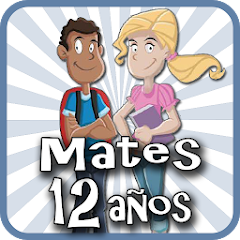# Matemáticas 12 años

4.0
772 reviews
100K+Everyone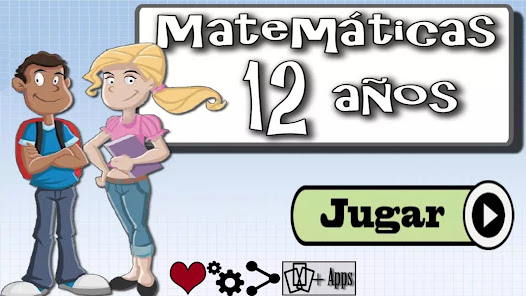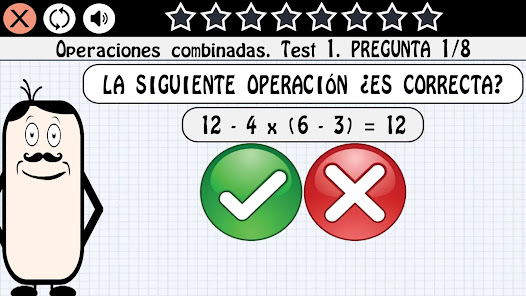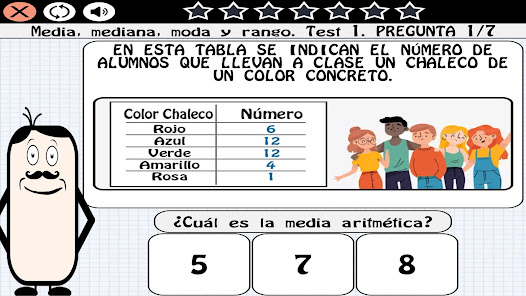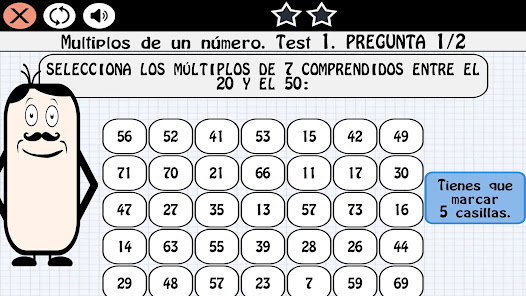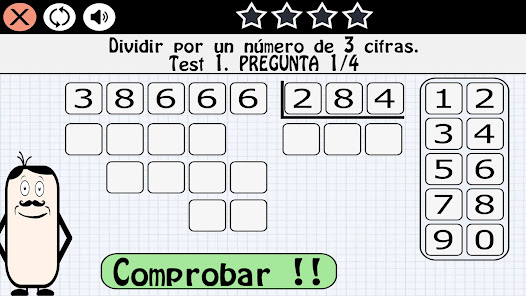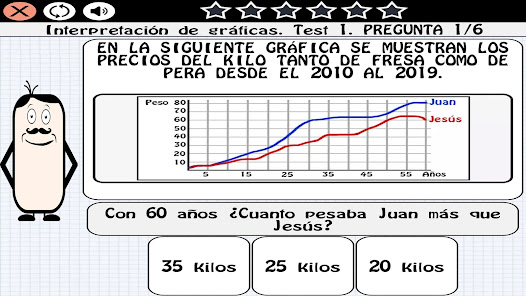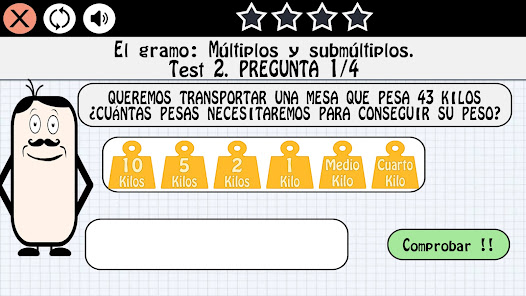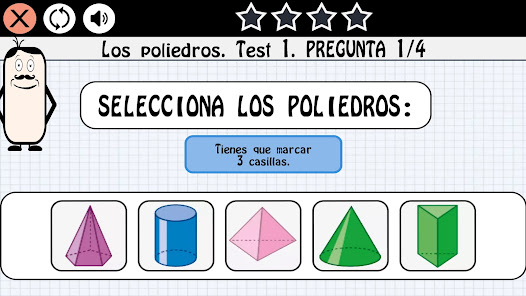Review the MATH syllabus for 12-year-old children with our fun application, completing the subject map until you take the final exam.

- Totally free.
- Specially designed for children of First ESO.
- 1000 questions.
- Final exam.
- Easy to play.
- Possibility to play without Internet.

Syllabus:
Natural numbers: Large numbers, Approximation by rounding, Properties of addition and relationship with subtraction, Multiplication by two digits, Multiplication problems, Dividing a 4-digit number by another 2, Dividing by a 3-digit number and Combined operations.
Powers and roots: Powers, squares and cubes, Powers of base 10, Polynomial decomposition of a number, Operations with powers, Square root, Exact roots and integer roots.
Divisibility: Multiples of a number, Least Common Multiple, Divisors of a number, Greatest Common Divisor, Prime and Composite Numbers.
Whole Numbers: Positive and Negative Numbers, Comparison and Ordering of Whole Numbers, Absolute Value and Opposite of an Integer, Addition of Whole Numbers, Whole Number Problems, Multiplication and Division of Whole Numbers, and Powers of Whole Numbers.
Decimal numbers: Decimal numbers, Comparison and ordering of decimals, Approximation by rounding decimals, Addition and subtraction of decimal numbers, Multiplication of decimal numbers and Division of decimal numbers.
The metric system: Submultiples of the meter, Multiples of the meter, Complex and incomplex expressions of measures of length, Problems of measures of length, The liter: Multiples and submultiples, Complex and incomplex expressions of measures of capacity, The gram: Multiples and submultiples, Complex and incomplex expressions of mass measurements, Surface Units and Volume Units.
Fractions: Fractions, The fraction of a quantity, Comparison of fractions and Equivalent Fractions.
Operations with fractions: Reduction to a common denominator, Addition and subtraction of fractions, Multiplication of fractions and Division of fractions.
Proportionality and percentages: Reduction to the unit, The rule of three, Percentages and Calculation of percentage of a quantity.
Equations: Equations and Equation Problems.
Perimeter and area of ​​plane figures: Areas of the square, rectangle, rhombus and rhomboid, Perimeter and area of ​​the triangle, Length of the circumference and Area of ​​the circle.
Geometric bodies: Prisms and pyramids, Polyhedrons and Bodies of revolution.
Angles: The measure of angles and Operations with measures of angles.
Graphs of functions: The Cartesian plane, Interpretation of graphs,
Statistics: Bar charts, Mean, Median, Mode, and Range.
Chance and probability: Sure, possible, impossible and Probability of an event.

Enjoy our application at no cost and reinforce your knowledge of mathematics for children of 12 years.
Updated on
Jan 17, 2023

## Data safety

Safety starts with understanding how developers collect and share your data. Data privacy and security practices may vary based on your use, region, and age. The developer provided this information and may update it over time.No data shared with third parties